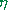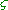SELF 32 S.B. Karavashkin and O.N. Karavashkina3. Einsteinian principle of relativity The first difficulty which Einstein encountered after he artificially introduced his L-postulate was to put it in agreement with the known Galilean principle of relativity. Einstein advanced not on the common, multiply checked way, how to reveal the regularities, analysing the amount of observable phenomena but on the way of adjustment of natural phenomena to his postulate. So he had to basically distort also the conception of relative motion in the nature. First of all it concerned the concept of simultaneity. “Before we created the theory of relativity, we premised that the concept of simultaneity has an absolute objective meaning even for events separated in space. This premise has been disproven by the discovery (for all that – discovery, not the borrow from the Maxwell theory! – Authors) of the law of light propagation. Actually, if the speed of light in a void appears to be the value independent of the choice (or, in other words, of the state of motion) of the inertial system to which it relates, we cannot give any absolute meaning to the concept of simultaneity of events separated by the spatial distance. Moreover, in each inertial frame there has to be established its special time. And if we do not use for the reference any system of coordinates (inertial system) (? – Authors), it is senseless to state that the events at different points of space occur simultaneously. Namely because of it the space and time merge in one four-dimensional continuum” [25, p. 243]. But as we showed above, long ago before relativists the classical physics has accounted the time that light needs to propagate – for example, in calculation of phase ratios in the interference. And Roemer accounted the time of signal travelling in the passing of information from remote. So relativistic claims that classical physics has incorrectly interpreted the simultaneity of events distanced in space were basically incorrect. The more that Einstein understood, the 4-D continuum is not a relativistic invention: “First of all we have to warn from an opinion that the four-dimension of reality has been first introduced by this theory (SR – Authors). Even in classical mechanics the ‘location’ of event is determined by four numbers: three spatial coordinates and one time coordinate; thus, the whole amount of physical ‘events’ is thought to be as if immersed into a 4-D continuous diversity (continuum)” [6, p. 753]. Another issue is that Einstein understood under the concept of relativity some basically other thing than classical physics has found. He tried to agree his discrepant L-postulate with the idea of relativity that in no way could be agreed with the non-physical postulating of the light speed constancy in all frames and, as we will show it below, gave absurd between-frames transformations. Basically, what is meant under the concept ‘simultaneity’? “Simultaneity is the coincidence in time of events separated from each other in space” [17, p. 339]. It follows from this definition that, in order we to be able, with account of finite speed of information propagation from one point of space to another, to detect the simultaneity of events, it is sufficient, the clocks at these points to be synchronised. So, if approaching formally, Einstein, denying simultaneity, rejected first of all the possibility of such synchronisation. At the same time Einstein had no intention to de-synch clocks, as without synchronisation he would be unable to derive his frame-to-frame transformation formulas, since he could not state surely that the body at the moment is at this point of this coordinate system. To point the instant, when the body was at the given point of space, he had to have at this point a clock synchronised with the clock in the coordinate origin. In other words, without synchronisation all regularities with which the physics operates become senseless. So in all his basic works, beginning with his first paper of 1905, the clocks in each frame were synch: “Let us imagine now that the space has been marked both in the resting frame K with the scale that is at rest in it and in the moving frame k with the scale moving with it and that, thus, we have yielded the coordinates x, y, z and relatively,,. Let through the resting clocks being at the resting frame and with help of light signals, by the technique pointed in the section 1 (that was specified in later works – Authors) we determine the time t of the resting frame for all points (! – Authors) of this last at which the clocks are present. Let further in the same way we determine the timefor the moving frame, for all points (! – Authors) of this frame in which there are the clocks resting with respect to this last, by the technique pointed in the section 1 between the points at which these clocks are located” [7, p. 13]. Not in vain in this citation we emphasised the non-specified Einstein’s definition of time synchronisation that he made in the section 1 of his work. In this section Einstein much carries on polemics about the everyday understanding of time, like “It can seem that all difficulties concerning the definition of ‘time’ can be got over if instead the word ‘time’ I write ‘the location of small arrow of my clock’…” [7, p. 9], but in the definition of synchronisation he confines himself only to the condition of commutative and distributive time intervals and to repeating the L-postulate. Of course, this is insufficient for synchronisation, and he wrote of it later: “Let us imagine that at many points there are located clocks resting with respect to the coordinate system. Let they all be equivalent, i.e. the difference of readings of two such clocks does not change. If now we imagine that these clocks are in some way synchronised, the amount of clocks located at quite small distances enables us to know the time of any pointed event with the help of nearest clock. But the ensemble of these readings of clocks yet does not give us ‘the time’ in the form necessary for physical purposes. Furthermore, we yet need to have a recipe how to check these clocks with each other” [27, p. 68]. Einstein gave later a more complete statement of synchronisation. “To get to know the time at each point of space, we can imagine the space filled with a vast number of clocks, and the clocks have to be absolutely alike… An ensemble of readings of all these clocks going in phase with each other will compose what we call the physical time” [16, p. 147–149]. And in the style typical for the whole his theory Einstein makes a distinction between his synchronisation and the methodology of classical physics in the same incorrect way: “To each event, except three spatial coordinates x, y, z , belongs the time coordinate t. It is premised that this last is measured by a clock (ideal periodic process) of infinitesimal time. We have to think this clock C resting at one of points of the coordinate system, for example, at the coordinate origin (x = y = z = 0). Then the time of event that occurred at the point P (x, y, z) is detected as the clock reading simultaneous with the event. Here the idea of ‘simultaneity’ was premised having the physical meaning without special definition. This is inaccurate (? – Authors), and it seems inoffensive only because with the help of light (whose speed is practically infinite from the view of everyday experience (? – Authors)) we, seemingly, would be able to directly establish the simultaneity of spatially separated events (which, as we showed it above, is an unprecedented slander of the methodology of classical physics – Authors). The special theory of relativity lifts this inaccuracy, introducing the physical definition of simultaneity with help of light signals. The time t of the event P is the reading of clock C at the moment of light signal arrival that came from event, except the time that the light signal needs to travel the distance to the clock. Such correction is based on the supposition (postulate) of the light speed constancy. This definition reduces the concept of simultaneity of spatially remote events to the concept of simultaneous events occurring at the same place (coincidence of events), namely – to the simultaneity of arrival of the light signal to C and reading of the time by the clock C” [26, p. 658–659]. As we can see, although in words Einstein as if disproved the simultaneity of events remote in space, saying it to be the merit of SR, none the less in each frame the simultaneity of events has remained (which is in full agreement with the concept of simultaneity in the given frame) and is determined in the same old way in which Roemer determined the light speed, observing the Jupiter moons as long ago as in 1678 – i.e. by way of subtracting from the time when the event occurred an interval of time needed this information to reach the observer. This made no hinder for Einstein to ascribe such technique to the defects of classical measurement techniques. And the main, in formulation of his technique to synchronise the clocks in one frame, Einstein needed not thinking the light speed infinite, just as supporters of classical physics had no need in it when they might not neglect the time during which the signal passed between the points at which the events occurred. For example, in calculation of interferometric patterns, the time dilations have been accounted in the same conventional way, and relativists did not and could not invent a better way.

Contents: / 28 / 29 / 30 / 31 / 32 / 33 / 34 / 35 / 36 / 37 / 38 / 39 / 40 / 41 / 42 /